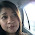### As easy as 123???

a) 10.84(cost) +10.05% (margin)= 11.93(SP)
b) 12.06(SP)-10.05% (margin)= 10.84(cost)

WHY? WHY? TELL ME WHY....沒有辦法做乖乖. :Pb) 想论证的 “同样的10.84为cost的话，而margin也是10.05%，为何SP会不一呢？？？”

SP - y% = x ?

a) What's my SP?
x + ( x * (y / 100) ) = SP

b) What's my cost (x)?
i) SP - ( x * (y / 100) ) = x

ii) SP = x + ( x * (y / 100) )
iii) SP = x + ( xy/ 100)
iv) SP = x(1 + (y/100))
v) SP/ (1+ (y/100)) = x

v) 11.93/ (1+ (10.05/100)) = 10.84

b 的程式，我做到iii后就不会了。后面是我求同事帮忙解出来的。我数学"肥佬"地。pepC said…

SP - y% = x ?（哈哈！你简直就是我肚子里的一条虫）pepC said…（刚读完了赖佩霞老师的书“回家”，也跑来“谈情说爱”了，惭愧，呵呵～～）pepC said…pepC said…